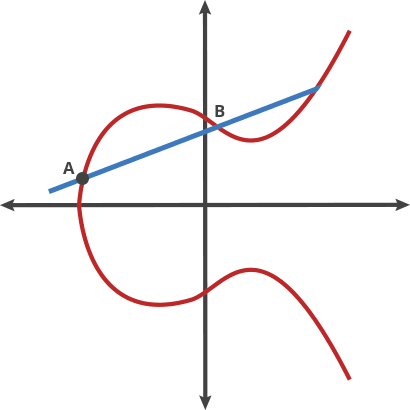# Why doesn't the formula work when checking two ECDSA signatures?

There are two generated ECDSA signatures

X - Private key

S  = ((Z  + (X * R)) / K) mod n

S = ((Z + (X * R)) / K) mod n


G - Base point, of order n;

R  = K  * G
R = K * G


Why is this formula not working?

C = K - K

R = R + (C * G)


Where exactly do I make a mistake?

• I don't understand your question. I think these formulas are correct. So what? In what way are they “not working”? – Gilles 'SO- stop being evil' Feb 26 '19 at 21:56
• Where exactly do I make a mistake? Explain to me please on my example: pastebin.com/qEgySGQ4 – Izi Tors Feb 27 '19 at 14:06

In ECDSA, the R value in the signature is not the elliptic curve point $$kG$$, but instead only its x-coordinate, that is, an integer.

So, what do you mean by R' + (C*G)? In particular, what do you mean by the operator +?

If you mean "the + is an integer modular addition, that is, we take the x-coordinate of the point $$CG$$, and then add it (modulo $$n$$) to $$R'$$", well, that'll give you an integer that's unrelated to $$R$$ (as $$R$$ and $$R'$$ are related as elliptic curve points not integers, and integer addition and elliptic curve operations have little to do with each other)

If you mean "the + is an elliptic curve addition, that is, we find one of the points with x-coordinate $$R'$$, and add it (using the elliptic curve addition operation) to the point $$C\cdot G$$), well, that'll work half the time; there are (usually) two elliptic curve points with x-coordinate $$R'$$, and it'll work if the one you pick just happens to be the original $$R'$$ point.

• Maybe I'm doing something wrong? For example, I want to take two ECDSA signatures: example – Izi Tors Feb 26 '19 at 23:22
• "I want to take two ECDSA signatures" and do what with them? – poncho Feb 26 '19 at 23:50
• I'm a newbie. Please explain to me by my example? I posted an example: pastebin.com/qEgySGQ4 I want to understand? – Izi Tors Feb 27 '19 at 19:19
• @IziTors: I believe you're being mislead by whatever software you are using; when it prints out a point (e.g. C*G), it lists it as a large integer. Actually, a point consists of both the X coordinate and a Y coordinate (as it is a solution to a specific cubic equation), and there's no obvious way to express both X and Y as a single integer. – poncho Feb 27 '19 at 19:35
• You can not describe this equation with the calculation of the value of pastebin.com/qEgySGQ4 – Izi Tors Feb 27 '19 at 19:48

Are you sure you understand how ECDSA or ECC work in terms of operations? The * and + operations are not actually multiplication and addition (in the last 2 equations). They are the ECC trapdoor function. You can see an animation of such a function here (it should be a gif):The * and + operations just mean applying the trapdoor function x times.

 R  = K  * G


Take the point G and apply the trapdoor function to it K times.

In ECC and ECDSA, a - operation (when refering to reversing the trapdoor function) is not possible in most scenarios, since that would mean reversing the trapdoor function, solving the Elliptic Curve Discrete Logarithm Porblem, making the cryptosystem obsolete (in most cases its not possible, but some attacks on weak curves exist).

You can find a bunch of python libraries to do these function multiplications like ECPy

• You can use a $-$, both on scalars and EC points. It is the discrete logarithm (the "division" of two points) that is intractable: "find $x$ given $X$,$G$ such that $X=x\cdot G$". – Ruben De Smet Feb 27 '19 at 13:17
• Yes, I meants doing a ‘-‘ operation on a point to reverse the trapdoor function and solve the ECDLP. – Tudor Feb 27 '19 at 13:19
• I'm a newbie. Please explain to me by my example? I posted an example: pastebin.com/qEgySGQ4 I want to understand? – Izi Tors Feb 27 '19 at 13:29
• Read my example and after you understand how a "+" or "*" work in ECDSA, read the wikipedia page for ECDSA. That is why I posted it, trying to explain these operations. – Tudor Feb 27 '19 at 13:32
• Here, for example, in my example, I minus: K = 247441A043B4DFD16A39D3834C596B351D1C3337BB48DC8D1113E9CAB2DE53A0 K = F9A0A1E22636D8662957B3D62066F182CD1FA37D3457E423CFC8EDB58793DED0 C = K - K I receive: C = D52C6041E281F894BF1DE052D40D864DB0037045790F0796BEB503EAD4B58B30 Then I get (C * G) = DDAAE15676A86275A5D793E5435329C5C3DFE8782E8CB825ED0D0E46E7AA1533 Right? – Izi Tors Feb 27 '19 at 13:58